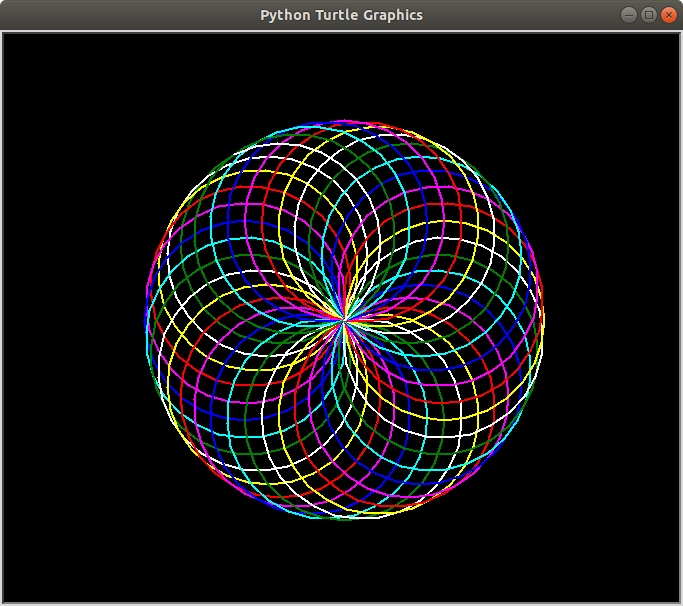Related Articles
Print a Spirograph using turtle in Python
• Last Updated : 28 Jul, 2020

Pre-requisites: Turtle Programming in Python

A spirograph is a very interesting geometrical figure which is often symmetrical to both the axes. It produces mathematical roulette curves of the variety technically known as hypotrochoids and epitrochoids. Here, we’ve used a range of colors to draw circles, you can use your combination as per your color choice.

Below is the implementation.

## Python3

 `# Import the turtle library for  ` `# drawing the required curve ` `import` `turtle as tt ` ` `  `# Set the background color as black, ` `# pensize as 2 and speed of drawing  ` `# curve as 10(relative) ` `tt.bgcolor(``'black'``) ` `tt.pensize(``2``) ` `tt.speed(``10``) ` ` `  `# Iterate six times in total ` `for` `i ``in` `range``(``6``): ` `   `  `      ``# Choose your color combination ` `    ``for` `color ``in` `(``'red'``, ``'magenta'``, ``'blue'``,  ` `                  ``'cyan'``, ``'green'``, ``'white'``, ` `                  ``'yellow'``): ` `        ``tt.color(color) ` `         `  `        ``# Draw a circle of choosen size, 100 here ` `        ``tt.circle(``100``) ` `         `  `        ``# Move 10 pixels left to draw another circle ` `        ``tt.left(``10``) ` `     `  `    ``# Hide the cursor(or turtle) which drew the circle ` `    ``tt.hideturtle()`

Output:Attention geek! Strengthen your foundations with the Python Programming Foundation Course and learn the basics.

To begin with, your interview preparations Enhance your Data Structures concepts with the Python DS Course.

My Personal Notes arrow_drop_up
Recommended Articles
Page :